# Looping Statements and Control Statements in Python

10 de Feb de 2020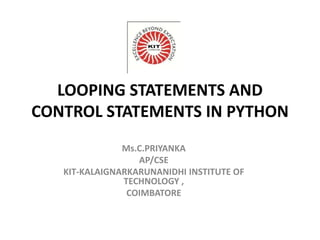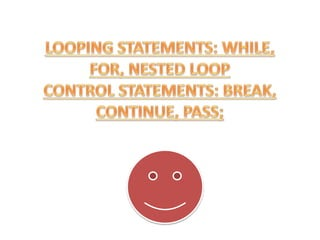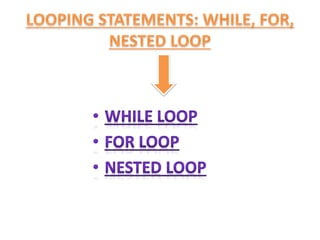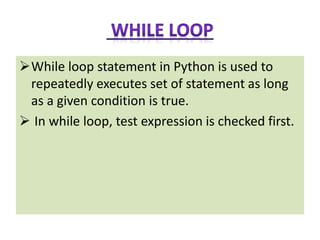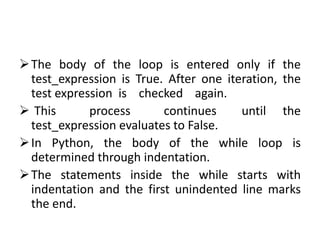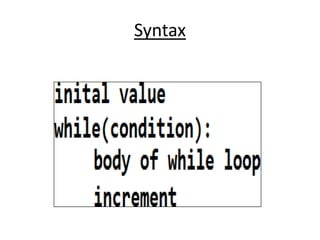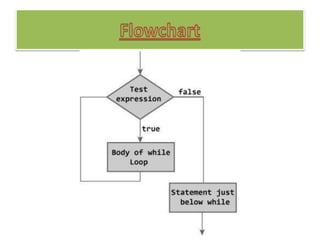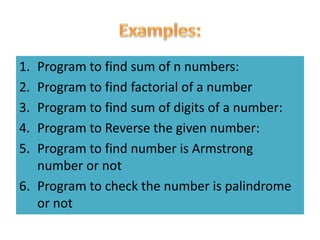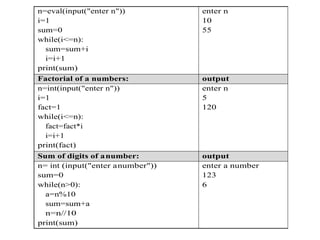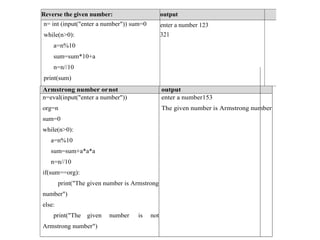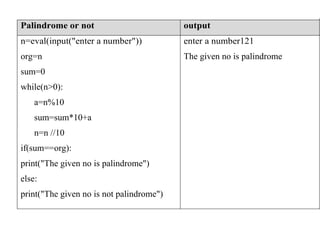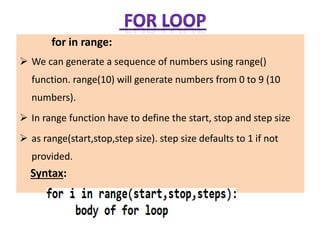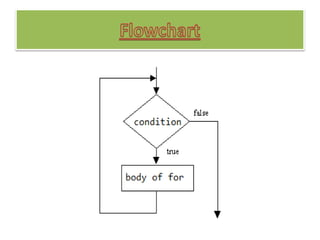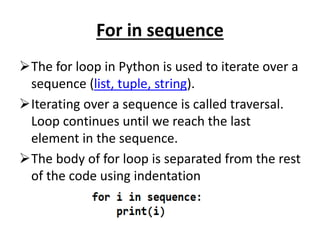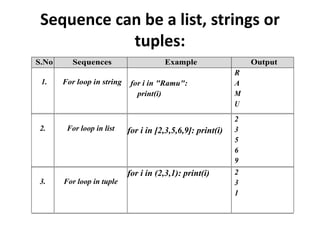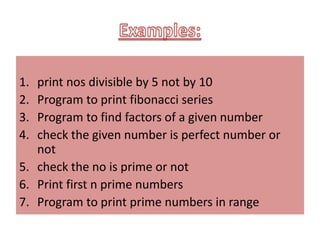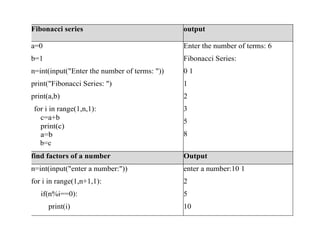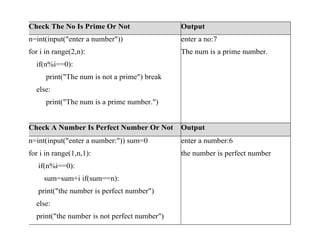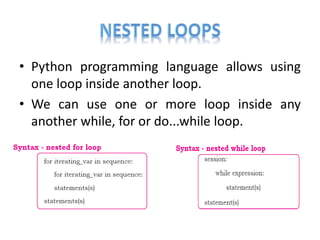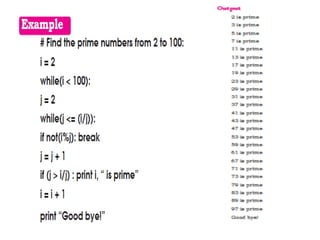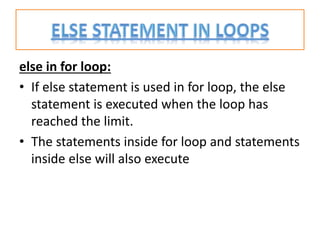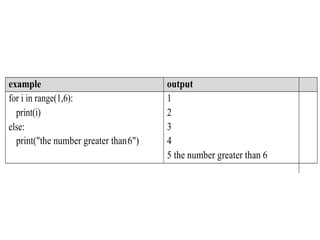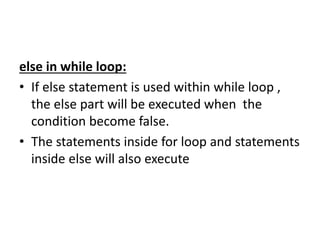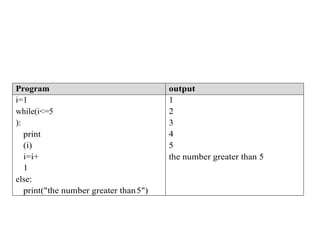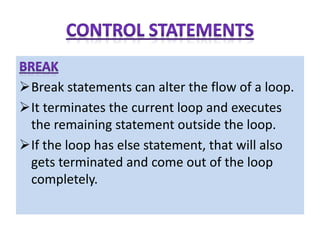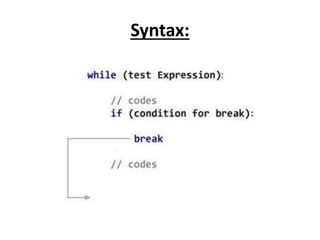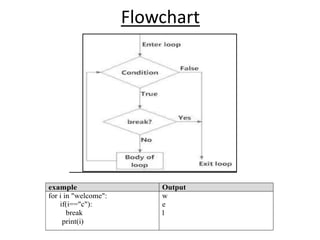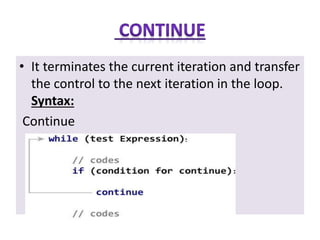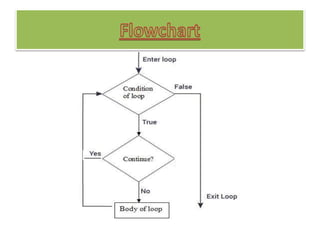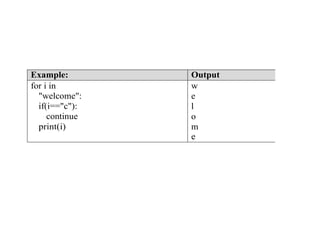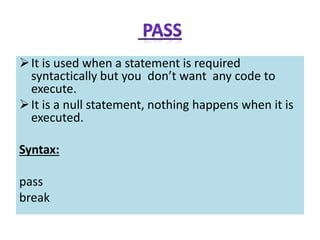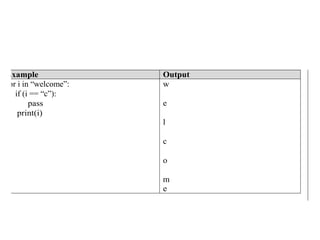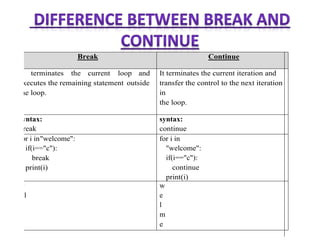1 de 33

### Looping Statements and Control Statements in Python

• 1. LOOPING STATEMENTS AND CONTROL STATEMENTS IN PYTHON Ms.C.PRIYANKA AP/CSE KIT-KALAIGNARKARUNANIDHI INSTITUTE OF TECHNOLOGY , COIMBATORE
• 4. While loop statement in Python is used to repeatedly executes set of statement as long as a given condition is true.  In while loop, test expression is checked first.
• 5. The body of the loop is entered only if the test_expression is True. After one iteration, the test expression is checked again.  This process continues until the test_expression evaluates to False. In Python, the body of the while loop is determined through indentation. The statements inside the while starts with indentation and the first unindented line marks the end.
• 6. Syntax
• 8. 1. Program to find sum of n numbers: 2. Program to find factorial of a number 3. Program to find sum of digits of a number: 4. Program to Reverse the given number: 5. Program to find number is Armstrong number or not 6. Program to check the number is palindrome or not
• 9. n=eval(input("enter n")) i=1 sum=0 while(i<=n): sum=sum+i i=i+1 print(sum) enter n 10 55 Factorial of a numbers: output n=int(input("enter n")) i=1 fact=1 while(i<=n): fact=fact*i i=i+1 print(fact) enter n 5 120 Sum of digits of anumber: output n= int (input("enter anumber")) sum=0 while(n>0): a=n%10 sum=sum+a n=n//10 print(sum) enter a number 123 6
• 10. Reverse the given number: output n= int (input("enter a number")) sum=0 while(n>0): a=n%10 sum=sum*10+a n=n//10 print(sum) enter a number 123 321 Armstrong number ornot output n=eval(input("enter a number")) enter a number153 org=n The given number is Armstrong number sum=0 while(n>0): a=n%10 sum=sum+a*a*a n=n//10 if(sum==org): print("The given number is Armstrong number") else: print("The given number is not Armstrong number")
• 11. Palindrome or not output n=eval(input("enter a number")) enter a number121 org=n The given no is palindrome sum=0 while(n>0): a=n%10 sum=sum*10+a n=n //10 if(sum==org): print("The given no is palindrome") else: print("The given no is not palindrome")
• 12. for in range:  We can generate a sequence of numbers using range() function. range(10) will generate numbers from 0 to 9 (10 numbers).  In range function have to define the start, stop and step size  as range(start,stop,step size). step size defaults to 1 if not provided. Syntax:
• 14. For in sequence The for loop in Python is used to iterate over a sequence (list, tuple, string). Iterating over a sequence is called traversal. Loop continues until we reach the last element in the sequence. The body of for loop is separated from the rest of the code using indentation
• 15. Sequence can be a list, strings or tuples: S.No Sequences Example Output 1. For loop in string for i in "Ramu": print(i) R A M U 2. For loop in list for i in [2,3,5,6,9]: print(i) 2 3 5 6 9 3. For loop in tuple for i in (2,3,1): print(i) 2 3 1
• 16. 1. print nos divisible by 5 not by 10 2. Program to print fibonacci series 3. Program to find factors of a given number 4. check the given number is perfect number or not 5. check the no is prime or not 6. Print first n prime numbers 7. Program to print prime numbers in range
• 17. Fibonacci series output a=0 b=1 n=int(input("Enter the number of terms: ")) print("Fibonacci Series: ") print(a,b) for i in range(1,n,1): c=a+b print(c) a=b b=c Enter the number of terms: 6 Fibonacci Series: 0 1 1 2 3 5 8 find factors of a number Output n=int(input("enter a number:")) for i in range(1,n+1,1): if(n%i==0): print(i) enter a number:10 1 2 5 10
• 18. Check The No Is Prime Or Not Output n=int(input("enter a number")) for i in range(2,n): if(n%i==0): print("The num is not a prime") break else: print("The num is a prime number.") enter a no:7 The num is a prime number. Check A Number Is Perfect Number Or Not Output n=int(input("enter a number:")) sum=0 for i in range(1,n,1): if(n%i==0): sum=sum+i if(sum==n): print("the number is perfect number") else: print("the number is not perfect number") enter a number:6 the number is perfect number
• 19. • Python programming language allows using one loop inside another loop. • We can use one or more loop inside any another while, for or do...while loop.
• 21. else in for loop: • If else statement is used in for loop, the else statement is executed when the loop has reached the limit. • The statements inside for loop and statements inside else will also execute
• 22. example output for i in range(1,6): print(i) else: print("the number greater than6") 1 2 3 4 5 the number greater than 6
• 23. else in while loop: • If else statement is used within while loop , the else part will be executed when the condition become false. • The statements inside for loop and statements inside else will also execute
• 24. Program output i=1 while(i<=5 ): print (i) i=i+ 1 else: print("the number greater than5") 1 2 3 4 5 the number greater than 5
• 25. Break statements can alter the flow of a loop. It terminates the current loop and executes the remaining statement outside the loop. If the loop has else statement, that will also gets terminated and come out of the loop completely.
• 26. Syntax:
• 27. Flowchart example Output for i in "welcome": if(i=="c"): break print(i) w e l
• 28. • It terminates the current iteration and transfer the control to the next iteration in the loop. Syntax: Continue
• 30. Example: Output for i in "welcome": if(i=="c"): continue print(i) w e l o m e
• 31. It is used when a statement is required syntactically but you don’t want any code to execute. It is a null statement, nothing happens when it is executed. Syntax: pass break
• 32. Example Output for i in “welcome”: if (i == “c”): pass print(i) w e l c o m e
• 33. Break Continue It terminates the current loop and executes the remaining statement outside the loop. It terminates the current iteration and transfer the control to the next iteration in the loop. syntax: break syntax: continue for i in"welcome": if(i=="c"): break print(i) for i in "welcome": if(i=="c"): continue print(i) w e l w e l m e